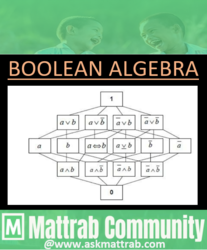Boolean Algebra - IntroductionIntroduction
The algebra of logic, which deals with the study of binary variables and logical operations, and also manipulates them is known as Boolean Algebra. This makes it possible to transform any logical statements into mathematical form in order to calculate the truth or falsity of related statements by using rules.
It was introduced by an English mathematician and logician George Boole. In Boolean algebra, variables are allowed to have only two values; ‘true’(T) and ‘false’ (F) usually written as 1 and 0 respectively. (also 'ON' for "True" & 'OFF' for "False" )

# ﻿Boolean Variables
The variables which have only two values either 0 or 1 are known as Boolean variables. It is also known as 'Logical Variable'.
Examples; A, B, X, Y are generally used as Boolean Variables.

# Boolean Values
The two logical values which represent either “True” or “False” values in Boolean algebra are called Boolean values. They are the possible values of Boolean Variables. "True" and "False" are the only Boolean Values which also be represented in following ways:
﻿True  ⇒ 1/ ON/ Yes/ High
False⇒ 0/OFF/ No/ Low
Generally, 1 and 0 are used as Boolean Values
[There is no hard and fast rule for choosing the Boolean Values.]

# Boolean Expressions
A statement/string which uses Boolean operators that represents logical variables and logical operations evaluated to give a logical value is called Boolean expression.
Example; AB +AB', A' + B , X+X'Y

# Boolean Operators
﻿The special symbols which indicates the operation to be carried out between two (set of)result are called Boolean Operators. There are three basic Boolean Operations:
1.  AND Operation → denoted by dot     ( . )
2.  OR Operation    → denoted by plus   ( + )
3.  NOT Operation → denoted by prime' )

# Logic Gates
A logic gate is an electronic circuit that operates on one or more input signals to produce an output signal. It is the basic component of a digital computer and is used for binary operation. The manipulation of Binary information is done by logic gate. It is the basic building block of computer and other digital devices. It is embodied in the Integrated Circuit (IC).